Smartick is a fun way to learn math!Nov11

# Multiplication of Decimals Using Money

Today we are going to better understand the multiplication of decimals by using a very common manipulative material: money. It is going to help us understand why multiplying by 10 moves the decimal position to the right.

### Equivalence of Bills or Coins with Numbers

First, we are going to establish the equivalence of each coin or bill with their written symbol, with the corresponding number.

Dollar bills are whole numbers and coins are decimal numbers. For example: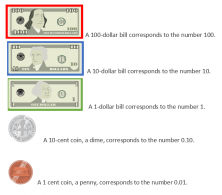This way, if we have:

• three 10 dollar bills
• five 1 dollar bills
• three 10 cent coins (dimes)
• and seven 1 cent coins (pennies)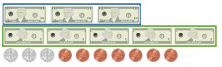There is a total of 35 DOLLARS AND 37 CENTS, or $35.37. ### Multiply Any Number by 10 To multiply a coin or dollar bill by 10, you just need to repeat it 10 times.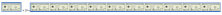Ten 10 dollar bills have the same value as one 100 dollar bill.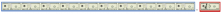Then,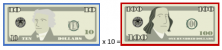Now, represented numerically: 10 x 10 = 100. The same thing happens with any coin: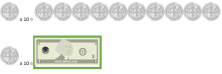0.1 x 10 = 1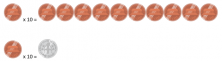0.01 x 10 = 0.1 As you can see, if we multiply any coin or bill by 10, the result is another coin or bill with a larger value. Exactly 10 times larger. As a result, the product of the multiplication is the same as moving the decimal point one space to the right. ### Multiplication of decimals with coins Once we know this we can go on to multiply a decimal by 10 and model it with coins. We are going to multiply 23.14 x 10. First, we will arrange the money vertically:$23.14.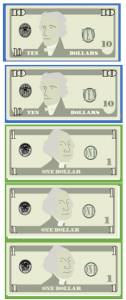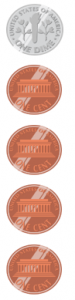Then we will repeat each coin and dollar bill 10 times: 23.14 x 10.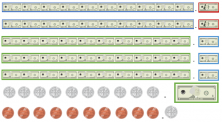Finally, we establish the equivalence of the corresponding bills and coins and we count: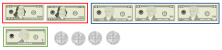23.14 x 10 = \$231.40

Using money as an example we can multiply decimals by 10 and understand why the decimal in the result moves to the right. You can use bills and coins, colored paper, or drawings to represent money. Now try some other multiplication problems, like 35.12 x 10, 16.43 x 10, or any that you can think of!

If you would like to know more about the multiplication of decimals, manipulative materials, and other elementary mathematics content, log on to Smartick and try it for free.

Fun is our brain’s favorite way of learning
Diane Ackerman
Smartick is a fun way to learn math
• 15 fun minutes a day